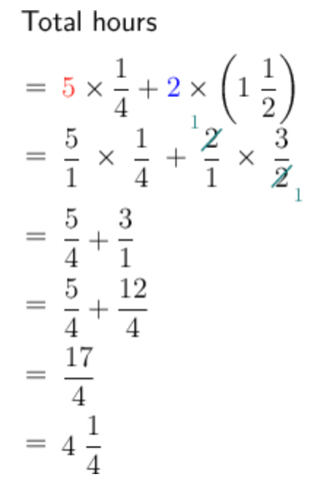Home Practice
For learners and parents For teachers and schools
Textbooks
Full catalogue
Pricing SupportLog in

We think you are located in United States. Is this correct?

# Solving problems

## Worked example 13.37: Using fractions to solve problems

Every weekday, Andiswa spends $$\frac{1}{4}$$ of an hour jogging when she gets back from school. On each day of the weekend, she spends $$1\frac{1}{2}$$ hours jogging. How many hours does she spend jogging in total each week?

### Summarise the given and the known information.

Given:

$$\frac{1}{4}$$ hour every weekday

$$1\frac{1}{2}$$ hour each day on the weekend

Known:

There are five weekdays and two days on the weekend.

### Choose the correct calculations.

We are looking for the total time spent jogging.

Multiply the weekday hours by five and the weekend hours by two, and then add them:

$$5 \times \frac{1}{4} =$$ total hours during the week

$$2 \times 1\frac{1}{2} =$$ total hours on the weekend

$$5 \times \frac{1}{4} + 2 \times 1\frac{1}{2} =$$ total time spent jogging

### Calculate the unknown amount.Therefore, Andiswa spends $$4\frac{1}{4}$$ hours jogging each week.

## Worked example 13.38: Using fractions to solve problems

Joshua is cooking a beef stew. The recipe calls for $$\frac{1}{2}$$ a tablespoon of spice mix per person. Today he is cooking for 9 people and he measures out the right amount of spice mix.

He then starts to think he might need a little extra stew, so he adds a bit more of each ingredient, including an extra $$1\frac{1}{4}$$ tablespoons of spice mix.

How many tablespoons of spice mix did he use in total?

### Summarise the given and the known information.

Given:

$$\frac{1}{2}$$ of a tablespoon of spice mix per person

There are $$9$$ people.

Added an extra $$1\frac{1}{4}$$ tablespoons of spice mix

### Choose the correct calculations.

We need to find the number of tablespoons of spice mix used in total.

Multiply the amount of spice mix per person by the number of people:

He must use $$\frac{1}{2}$$ a tablespoon of spice mix per person plus $$1\frac{1}{4}$$ tablespoons. Joshua is cooking for $$9$$ people.

$9 \times \frac{1}{2} + 1\frac{1}{4}$

### Calculate the unknown amount.

\begin{align} 9 \times \frac{1}{2} + 1\frac{1}{4} &= \frac{9}{1} \times \frac{1}{2} + \frac{5}{4} \\ &= \frac{9}{2} + \frac{5}{4} \\ &= \frac{18}{4} + \frac{5}{4} \\ &= \frac{23}{4} \\ &= 5\frac{3}{4} \end{align}

Therefore, Joshua must use $$5\frac{3}{4}$$ tablespoons of spice mix.

temp text